##CS 660: Combinatorial Algorithms Dynamic Lists

[To Lecture Notes Index]
San Diego State University -- This page last updated Sept. 5, 1995## References

Hester, James and Hirschberg, Daniel, "Self-Organizing Linear Search", Computing Surveys, 17(3):295-311, September 1985.

## Searching

Search for x in a list of n data itemsStandard Solution

* Sort the list of data items (or create a binary search tree)
Cost for general list is Theta(nlg(n))

* Now search for x
Average and worst case cost is Theta(lg(n))

Hidden Assumptions

* We will perform more than one search
Number of searches should be Omega(lg(n))

* All items in the list will be searched for with nearly the same frequency
Contrived Example

Assume we have a list of n items: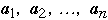The a's are ordered by frequency of access

Probability of accessing item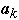: P() =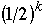Probability of looking for an item not in the list is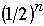Average cost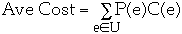U = set of all possible events
P(e) = probability of event e
C(e) = cost of event e

We have:What is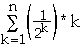?

We have: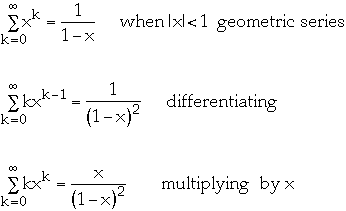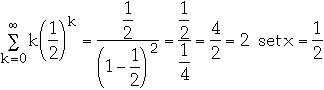SoThus Ave Cost =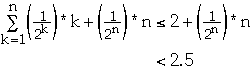## Self-Organizing Linear Search

Why linear search?

* Simple to code
```	location = -1
for (K = 0; K < n; K++)
if ( data[K].key == X)  {
location = K;
break;
}
```

* Requires minimal space

Organizing the list

Assume we have a list of n items:Probability of accessing item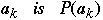* Optimal Static Ordering

* Move-to-front

* Transpose

Optimal Static Ordering

Assume P(ak) is known for all k in advance

Order items in decreasing probability

Example: 2.0 average comparisons
Let P(a) = .2
P(b) = .4 P(c) = .3 P(d) = .1
Optimal static ordering
b, c, a, d

Move-to-front

Start with any initial ordering

When item is accessed move it to the front of the list

Example: 2.2 average comparisons
```	a,	b,	c,	d	start order
b,	a,	c,	d	accessed b	2 comparisons
b,	a,	c,	d	accessed b	1
a,	b,	c,	d	accessed a	2
c,	a,	b,	d	accessed c	3
a,	c,	b,	d	accessed a	2
c,	a,	b,	d	accessed c	2
c,	a,	b,	d	accessed c	1
d,	c,	a,	b	accessed d	4
b,	d,	c,	a	accessed b	4
b,	d,	c,	a	accessed b	1
```
Transpose

Start with any initial ordering

When item is accessed move it forward one location

Example: 2.3 average comparisons
```	a,	b,	c,	d	start order
b,	a,	c,	d	accessed b	2 comparisons
b,	a,	c,	d	accessed b	1
a,	b,	c,	d	accessed a	2
a,	c,	b,	d	accessed c	3
a,	c,	b,	d	accessed a	1
c,	a,	b,	d	accessed c	2
c,	a,	b,	d	accessed c	1
c,	a,	d,	b	accessed d	4
c,	a,	b,	d	accessed b	4
c,	b,	a,	d	accessed b	3
```

### General Information and Restrictions

Permutation algorithms
Algorithm used to rearrange list after accessing a record

Restrictions
Only consider permutation algorithms that move accessed item forward in the list
Will not search for items not in the list
All items will be searched at least once
Time required by any execution of the permutation algorithm is never more than a constant times the time required for the search immediately before that execution.
Example
Given the listAccessing second item requires two comparisons so permutation algorithm can take c*2 time units
Accessing the last item requires two comparisons so permutation algorithm can take c*n time units

Measures of Performancethe search sequence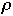[k] the item to be searched for on the k'th access(, k) be the state of the list after the first k accesses from [[rho]](, k)r location in the list of item r after the first k accesses from [[rho]]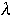=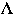(, 0) the initial configuration of the list

Cost of a permutationfor a given l and r is the average cost per access in terms of the number of probes required to find the accessed record and the work required to permute the records afterwardsAsymptotic Cost
Average cost over allandfor a givenUsually restrictto make analysis possible

### Zipf's Law

Zipf noticed that in English the frequency of word usage follows: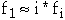where fi denotes the frequency of the ith most frequent word

Zipfian Probability Distribution:

Assume we have a list of n items:The a's are ordered by frequency of access

Probability of accessing itemis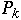andThenBut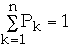so we have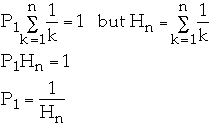Zipfian Probability Distribution
Let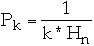for k = 1, 2, ..., n

where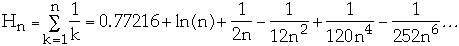Pk
```n = 2
k	1	2
Pk	0.6667	0.3333

n = 3
k	1	2	3
Pk	0.5455	0.2727	0.1818

n = 4
k	1	2	3	4
Pk	0.48	0.24	0.16	0.12

n = 5
k	1	2	3	4	5
Pk	0.438	0.219	0.146	0.109	0.0876

n = 6
k	1	2	3	4	5	6
Pk	0.408	0.204	0.136	0.102	0.0816	.0680
```

How to Implement Zipf's Distribution

LetMethod 1

If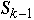<= rand() <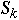then return k
Method 2

### Lotka's Law

The number of papers in a given journal written by the same author follows an inverse square distribution.

Let n be the total number of authors who published at least one paper in a given journal.

The probability that a randomly chosen author contributed exactly k papers is given by: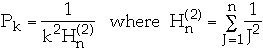```n = 2
k	1	2
Pk	0.800	0.200

n =3
k	1	2	3
Pk	0.734	0.183	0.081

n =4
k	1	2	3	4
Pk	0.702	0.175	0.078	0.043

n =5
k	1	2	3	4	5
Pk	0.683	0.170	0.075	0.042	0.027

n =6
k	1	2	3	4	5	6
Pk	0.670	0.167	0.074	0.041	0.026	0.018
```

### 80% - 20% Rule

"80% of the transactions are on the most 20% of the records, and so on recursively"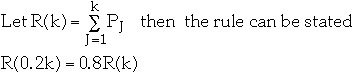When n = 5*L we have: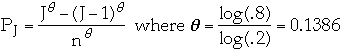```n = 2
k	1	2
Pk	0.908	0.100

n = 3
k	1	2	3
Pk	0.858	0.100	0.063

n = 4
k	1	2	3	4
Pk	0.825	0.100	0.063	0.047

n = 5
k	1	2	3	4	5
Pk	0.800	0.100	0.063	0.047	0.038
```

80% - 20% Rule

Knuth claims we can approximate this by:```n = 2
k	1	2
Pk	0.644	0.355

n = 3
k	1	2	3
Pk	0.515	0.283	0.200

n = 4
k	1	2	3	4
Pk	0.446	0.245	0.173	0.135

n = 5
k	1	2	3	4	5
Pk	0.401	0.220	0.155	0.121	0.100

n = 6
k	1	2	3	4	5	6
Pk	0.369	0.203	0.143	0.111	0.092	0.078
```

### Convergence to Steady State

Steady State
Further permutations are not expected to change the expected search time significantly
Locality
Subsequences of [[rho]] may have relative frequencies of access that are drastically different from the overall relative frequencies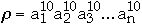Total Number of Comparisons in searchWorst Case
```	n	Move-to-Front	BST
7	112	170
15	360	490
31	1240	1290
63	4536	3210
```

Measures of Convergence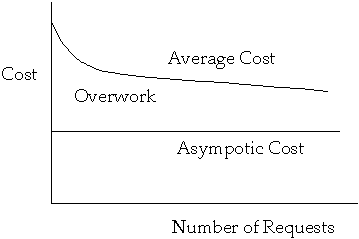Relative Measurements
Optimal Static Ordering - items are ordered by static probability of access and are not movedTotal Number of Comparisons in searchWorst Case
```	n	Move-to-Front	OSO
7	112	210
15	360	1050
31	1240	4650
```

### Known Algorithms and Analysis

Move-to-front
Assume Zipf distribution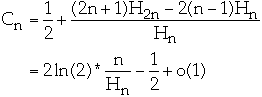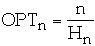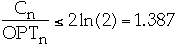TransposeCount
approaches optimal static ordering

Comparisons between Algorithms

No Optimal Memoryless algorithm

Asymptotic Cost
Move-to-front asymptotic cost at most twice asymptotic cost of the optimal static ordering
Asymptotic cost of transpose is <= asymptotic cost of move-to-front
Count is asymptotically equal to optimal static ordering
Worst Case
Move-to-front and count at most twice the worst case of the optimal static ordering
Transpose can be far worse
Moving a record any fraction of the distance to the front of the list will be no more than a constant times the optimal off-line algorithm
The constant is inversely proportional to the fraction of the total distance moved